×#### Thank you for registering.

One of our academic counsellors will contact you within 1 working day.

Click to Chat

1800-1023-196

+91-120-4616500

CART 0

• 0

MY CART (5)

Use Coupon: CART20 and get 20% off on all online Study Material

ITEM
DETAILS
MRP
DISCOUNT
FINAL PRICE
Total Price: Rs.

There are no items in this cart.
Continue Shopping• Complete JEE Main/Advanced Course and Test Series
• OFFERED PRICE: Rs. 15,900
• View Details

```Chapter 16: Circles Exercise – 16.4

Question: 1

In figure, O is the centre of the circle. If ∠APB = 50°, find ∠AOB and ∠OAB.Solution:

∠APB = 50°

By degree measure theorem

∠AOB = 2∠APB

⇒ ∠APB = 2 × 50° = 100° since OA = OB [Radius of circle]

Then ∠OAB = ∠OBA [Angles opposite toequalsides]

Let ∠OAB = x

In ΔOAB, by angle sum property

∠OAB + ∠OBA + ∠AOB = 180°

⟹ x + x + 100° = 180°

⟹ 2x = 180° - 100°

⟹ 2x = 80°

⟹ x = 40°

∠OAB = ∠OBA = 40°

Question: 2

In figure, it is given that O is the centre of the circle and ∠AOC = 150°. Find ∠ABC.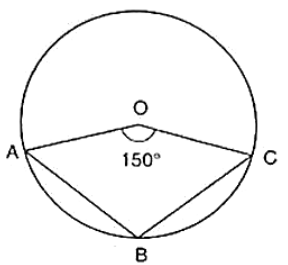Solution:

∠AOC = 150°

∴ ∠AOC + reflex ∠AOC = 360° [Complex angle]

⇒ 150° + reflex ∠AOC = 360°

⇒ reflex ∠AOC = 360° − 150°

⇒ reflex ∠AOC = 210°

⇒ 2∠ABC = 210° [By degree measure theorem]

⇒ ∠ABC = 210°/2 = 105°

Question: 3

In figure, O is the centre of the circle. Find ∠BAC.Solution:

We have ∠AOB = 80°

And ∠AOC = 110°

Therefore, ∠AOB + ∠AOC + ∠BOC = 360° [Complete angle]

⇒ 80° + 100° + ∠BOC = 360°

⇒ ∠BOC = 360° − 80° − 110°

⇒ ∠BOC = 170°

By degree measure theorem

∠BOC = 2∠BAC

⇒ 170° = 2∠BAC

⇒ ∠BAC = 170°/2 = 85°

Question: 4

If O is the centre of the circle, find the value of x in each of the following figures.

(i)(ii)(iii)(iv)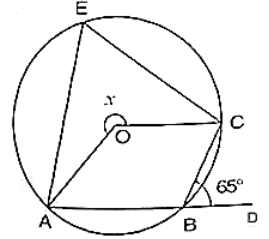(v)(vi)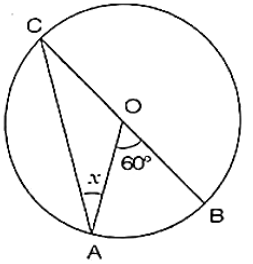(vii)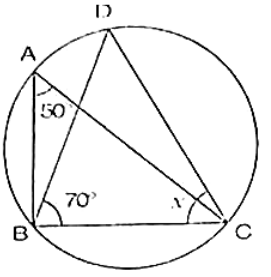(viii)(ix)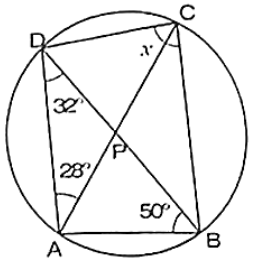(x)(xi)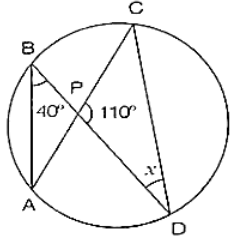(xii)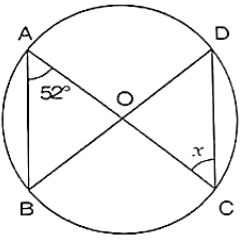Solution:

(i) ∠AOC = 135°

∴ ∠AOC + ∠BOC = 180° [Linear pair of angles]

⇒ 135° + ∠BOC = 180°

⇒ ∠BOC = 180° − 135°

⇒ ∠BOC = 45°

By degree measure theorem

∠BOC = 2∠CPB

⇒ 45° = 2x

⇒ x = 45°/2 = 22½°

(ii) We have ∠ABC = 40°

∠ACB = 90° [Angle in semi circle]

In ΔABC, by angle sum property

∠CAB + ∠ACB + ∠ABC = 180°

⇒ ∠CAB + 90° + 40° = 180°

⇒ ∠CAB = 180° − 90° − 40°

⇒ ∠CAB = 50°

Now, ∠CDB = ∠CAB [Angle is same in segment]

⇒ x = 50°

(iii) We have

∠AOC = 120° By degree measure theorem.

∠AOC = 2∠APC

⇒ 120° = 2∠APC

⇒ ∠APC = 120°/2 = 60°

∠APC + ∠ABC = 180° [Opposite angles of cyclic quadrilaterals]

⇒ 60° + ∠ABC = 180°

⇒ ∠ABC = 180° − 60°

⇒ ∠ABC = 120°

∴ ∠ABC + ∠DBC = 180° [Linear pair of angles]

⇒ 120 + x = 180°

⇒ x = 180° − 120° = 60°

(iv)  We have

∠CBD = 65°

∴ ∠ABC + ∠CBD = 180° [Linear pair of angles]

⇒ ∠ABC = 65° = 180°

⇒ ∠ABC = 180° − 65° = 115°

∴ reflex ∠AOC = 2∠ABC [By degree measure theorem]

⇒ x = 2 × 115°

⇒ x = 230°

(v) We have

∠OAB = 35° Then,

∠OBA = ∠OAB = 35° [Angles opposite to equal radii]

In ΔAOB, by angle sum property

⇒∠AOB + ∠OAB + ∠OBA = 180°

⇒ ∠AOB + 35° + 35° = 180°

⇒ ∠AOB = 180° −35° − 35° = 110°

∴ ∠AOB + reflex ∠AOB = 360° [Complexangle]

⇒ 110° + reflex ∠AOB = 360°

⇒ reflex ∠AOB = 360° − 110° = 250°

By degree measure theorem reflex

∠AOB = 2∠ACB

⇒ 250° = 2x

⇒ x = 250°/2 = 125°

(vi) We have

∠AOB = 60° By degree measure theorem reflex

∠AOB = 2∠ACB

⇒ 60° = 2∠ACB

⇒ ∠ACB = 60°/2 = 30° [Angles opposite to equal radii]

⇒ x = 30°.

(vii) We have

∠BAC = 50° and ∠DBC = 70°

∴ ∠BDC = ∠BAC = 50° [Angle in same segment]

In ΔBDC, by angle sum property

∠BDC + ∠BCD + ∠DBC = 180°

⇒ 50° + x + 70° = 180°

⇒ x = 180° − 50° − 70° = 60°

(viii) We have

∠DBO = 40° and ∠DBC = 90° [Angle in a semicircle]

⇒ ∠DBO + ∠OBC = 90°

⇒ 40° + ∠OBC = 90°

⇒ ∠OBC = 90° − 40° = 50° By degree measure theorem

∠AOC = 2∠OBC

⇒ x = 2 × 50° = 100°

(ix)  In ΔDAB, by angle sum property

∠ADB + ∠DAB + ∠ABD = 180°

⇒ 32° + ∠DAB + 50° = 180°

⇒ ∠DAB = 180° − 32° − 50°

⇒ ∠DAB = 98°

Now, ∠OAB + ∠DCB = 180° [Opposite angles of cyclic quadrilateral]

⇒ 98° + x = 180°

⇒ x = 180° − 98° = 82°

(x) We have,

∠BAC = 35°

∠BDC = ∠BAC = 35° [Angle in same segment]

In ΔBCD, by angle sum property

∠BDC + ∠BCD + ∠DBC = 180°

⇒ 35° + x + 65° = 180°

⇒ x = 180° − 35° − 65° = 80°

(xi) We have

∠ABD = 40°

∠ACD = ∠ABD = 40° [Angle in same segment]

In ΔPCD, by angle sum property

∠PCD + ∠CPO + ∠PDC = 180°

⇒ 40° + 110° + x = 180°

⇒ x = 180° − 150°

⇒ x = 30°

(xii) Given that,

∠BAC = 52°

Then ∠BDC = ∠BAC = 52° [Angle in same segment]

Since OD = OC

Then ∠ODC = ∠OCD [Opposite angle to equal radii]

⇒ x = 52°

Question: 5

O is the circumference of the triangle ABC and OD is perpendicular on BC. Prove that ∠BOD = ∠A.

Solution:

Given O is the circum centre of triangle ABC and OD⊥BC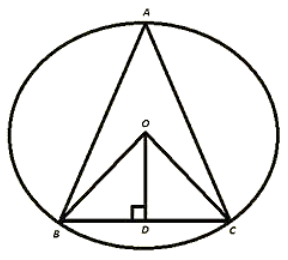To prove ∠BOD = 2∠A

Proof:

In ΔOBD and ΔOCD

∠ODB = ∠ODC [Each 90°]

OB = OC [Radius of circle]

OD = OD [Common]

Then ΔOBD ≅ ΔOCD    [By RHS Condition].

∴ ∠BOD = ∠COD  .... (i)   [PCT].

By degree measure theorem

∠BOC = 2∠BAC

⇒ 2∠BOD = 2∠BAC [By using (i)]

⇒ ∠BOD = ∠BAC.

Question: 6

In figure, O is the centre of the circle, BO is the bisector of ∠ABC. Show that AB = AC.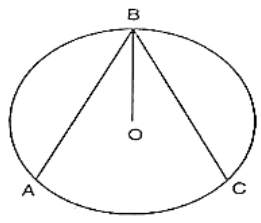Solution:

Given, BO is the bisector of ∠ABC

To prove AB = BC

Proof:

Since, BO is the bisector of ∠ABC.

Then, ∠ABO = ∠CBO ... (i)

Since, OB = OA         [Radius of circle]

Then, ∠ABO = ∠DAB... (ii)      [opposite angles to equal sides]

Since OB = OC                       [Radius of circle]

Then, ∠OAB = ∠OCB... (iii) [opposite angles to equal sides]

Compare equations (i), (ii) and (iii)

∠OAB = ∠OCB ... (iv)

In ΔOAB and ΔOCB

∠OAB = ∠OCB            From (iv)]

∠OBA = ∠OBC     [Given]

OB = OB                   [Common]

Then, ΔOAB ≅ ΔOCB [By AAS condition]

∴  AB = BC [CPCT]

Question: 7

In figure, O is the centre of the circle, then prove that ∠x = ∠y + ∠z.Solution:

We have,

∠3 = ∠4 [Angles in same segment]

∴ ∠x = 2∠3 [By degree measure theorem]

⇒ ∠x = ∠3 + ∠3

⇒ ∠x = ∠3 + ∠4 ... (i) [∠3 = angle 4]

But ∠y = ∠3 + ∠1 [By exterior angle property]

⇒ ∠3 = ∠y − ∠1 .... (ii)

from (i) and (ii)

∠x = ∠y − ∠1 + ∠4

⇒ ∠x = ∠y + ∠4 − ∠1

⇒ ∠x = ∠y + ∠z + ∠1 − ∠1 [By exterior angle property]

⇒ ∠x = ∠y + ∠z

Question: 8

In figure, O and O' are centers of two circles intersecting at B and C. ACD is a straight line, find x.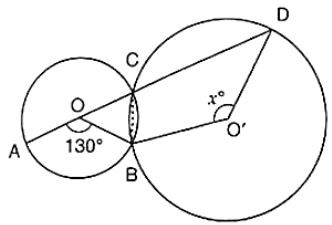Solution:

By degree measure theorem

∠AOB = 2∠ACB

⇒ 130° = 2∠ACB

⇒ ∠ACB = 130°/2 = 65°

∴ ∠ACB + ∠BCD = 180° [Liner a pair of angles]

⇒ 65° + ∠BCD = 180°

⇒ ∠BCD = 180° − 65° = 115° By degree measure theorem reflex

∠BOD = 2∠BCD

⇒ reflex ∠BOD = 2 × 115° = 230°

Now, reflex ∠BOD + ∠BO′D = 360° [Complex angle]

⇒ 230° + x = 360°

⇒ x = 360° − 230°

∴ x = 130°

Question: 9

In figure, O is the centre of a circle and PQ is a diameter. If

∠ROS = 40°, find ∠RTS.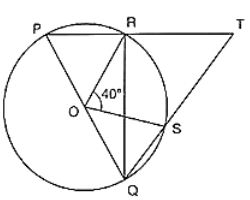Solution:

Since PQ is diameter

Then,

∠PRQ = 90° [Angle in semicircle]

∴ ∠PRQ + ∠TRQ = 180° [Linear pair of angle]

900 + ∠TRQ = 180°

∠TRQ = 180° − 90° = 90°.

By degree measure theorem

∠ROS = 2∠RQS

⇒ 40° = 2∠RQS

⇒ ∠RQS = 40°/2 = 20°

In ΔRQT, by Angle sum property

∠RQT + ∠QRT + ∠RTS = 180°

⇒ 20° + 90° + ∠RTS = 180°

⇒ ∠RTS = 180° − 20° − 90° = 70°

Question: 10

In figure, if ∠ACB = 40°, ∠DPB = 120°, find ∠CBD.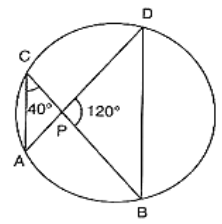Solution:

We have,

∠ACB = 40°; ∠DPB = 120°

∴ ∠APB = ∠DCB = 40° [Angle in same segment]

In ΔPOB, by angle sum property

∠PDB + ∠PBD + ∠BPD = 180°

⇒ 40° + ∠PBD + 120° = 180°

⇒ ∠PBD = 180° − 40° − 120°

⇒ ∠PBD = 20°

∴ ∠CBD = 20°

Question: 11

A chord of a circle is equal to the radius of the circle. Find the angle subtended by the chord at a point on the minor arc and also at a point on the major arc.

Solution:

We have,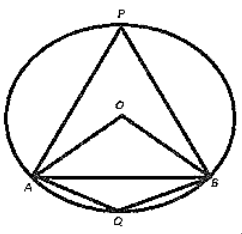Radius OA = Chord AB

⟹ OA = OB = AB

Then triangle OAB is an equilateral triangle.

∴ ∠AOB = 60° [one angle of equilateral triangle]

By degree measure theorem

∠AOB = 2∠APB

⇒ 60° = 2∠APB

⇒ ∠APB = 60°/2 = 30°

Now, ∠APB + ∠AQB = 180° [opposite angles of cyclic quadrilateral]

⇒ 300 + ∠AQB = 180°

⇒ ∠AQB = 180° − 30° = 150°.

Therefore, Angle by chord AB at minor arc = 150°

Angle by chord AB at major arc = 30°
```### Course Features

• 728 Video Lectures
• Revision Notes
• Previous Year Papers
• Mind Map
• Study Planner
• NCERT Solutions
• Discussion Forum
• Test paper with Video Solution